# 5.3D: $$BF_3$$

$$\newcommand{\vecs}{\overset { \rightharpoonup} {\mathbf{#1}} }$$ $$\newcommand{\vecd}{\overset{-\!-\!\rightharpoonup}{\vphantom{a}\smash {#1}}}$$$$\newcommand{\id}{\mathrm{id}}$$ $$\newcommand{\Span}{\mathrm{span}}$$ $$\newcommand{\kernel}{\mathrm{null}\,}$$ $$\newcommand{\range}{\mathrm{range}\,}$$ $$\newcommand{\RealPart}{\mathrm{Re}}$$ $$\newcommand{\ImaginaryPart}{\mathrm{Im}}$$ $$\newcommand{\Argument}{\mathrm{Arg}}$$ $$\newcommand{\norm}{\| #1 \|}$$ $$\newcommand{\inner}{\langle #1, #2 \rangle}$$ $$\newcommand{\Span}{\mathrm{span}}$$ $$\newcommand{\id}{\mathrm{id}}$$ $$\newcommand{\Span}{\mathrm{span}}$$ $$\newcommand{\kernel}{\mathrm{null}\,}$$ $$\newcommand{\range}{\mathrm{range}\,}$$ $$\newcommand{\RealPart}{\mathrm{Re}}$$ $$\newcommand{\ImaginaryPart}{\mathrm{Im}}$$ $$\newcommand{\Argument}{\mathrm{Arg}}$$ $$\newcommand{\norm}{\| #1 \|}$$ $$\newcommand{\inner}{\langle #1, #2 \rangle}$$ $$\newcommand{\Span}{\mathrm{span}}$$$$\newcommand{\AA}{\unicode[.8,0]{x212B}}$$

The Valence Bond Theory is usually represented by the Lewis dot models. Boron is an unusual molecule because it does not follow the octet rule by having eight valence electrons around the boron atom. BF3 has single bonds between the boron atom and the fluorine atoms and contains no double bonds and an empty p orbital (figure 3). This is not predicted by the valence bond theory because it does not allow for any empty orbitals.

Valence bond theory and how it fails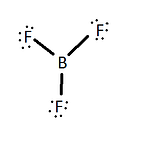figure 1

Valence Bond Theory states that when a half filled orbital, in contact with another half filled orbital, will hybridize to form a more stable bonding orbital. According to the Valence Bond Theory, the lone pair of one of the fluorine atoms should overlap to form a pi bond with the boron atom through a double bond in order to complete an octet on the boron atom (figure 2), but this does not occur.

There are two major reasons why there is no pi bond between any of the F atoms and B atoms: electronegativity and separation of charge. If one were to write out formal charges of the molecule without the pi bond, they will find that the formal charges on all of the atoms are 0. The boron shares it's 3 valence electrons with the fluorine creating a total of 6 electrons shared with the fluorine, making it's formal charge 0. Each fluorine shares one of it's valence electrons creating a total of 2 shared electrons with boron and has 6 more electrons to themselves, making their formal charges also 0 (figure 1).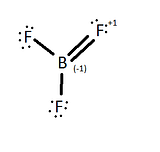figure 2

If one of the fluorines were to form a pi bond with the boron, then the fluorine, which is the most electronegative element, will have an oxidized state and a formal charge of +1 and, consequently the boron atom would have a formal charge of -1. Although this still leaves the BF3 molecule to have an overall formal charge of 0, the most stable form would be BF3 with no pi bonds because each of the atom's formal charge is 0. The most stable state is to leave the boron with an empty p orbital.

The empty p orbital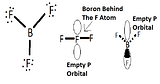figure 3

BF3 is a planar molecule because it does not have a lone pair, which makes it have a trigonal planar geometry. BF3 is considered a Lewis acid because it accepts electrons at its empty p orbital.

Examples of reactivity of the empty p orbital

The most common reactions with involving BF3 is to form complexes with ethers.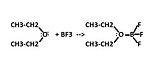figure 4

Other molecules like BF3

Carbenes are molecules that are used for different organic synthesis reactions. They typically involve a carbon with a lone pair and 2 bonded halides (like bromide). It also includes this empty p orbital and is fairly reactive.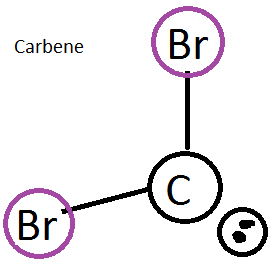figure 5

5.3D: $$BF_3$$ is shared under a CC BY-NC-SA 4.0 license and was authored, remixed, and/or curated by LibreTexts.